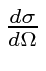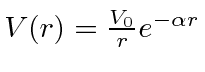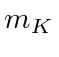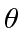## Sample Test Problems

1. Calculate the differential cross section,, for high energy scattering of particles of momentum p, from a spherical shell delta functionAssume that the potential is weak so that perturbation theory can be used. Be sure to write your answer in terms of the scattering angles.
2. Assume that a heavy nucleus attractsmesons with a weak Yakawa potential. Calculate the differential cross section,, for scattering high energymesons (mass) from that nucleus. Give your answer in terms of the scattering angle.

Jim Branson 2013-04-22